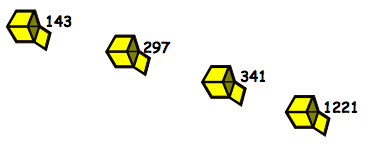#### You may also like### Consecutive Numbers

An investigation involving adding and subtracting sets of consecutive numbers. Lots to find out, lots to explore.### Roll These Dice

Roll two red dice and a green dice. Add the two numbers on the red dice and take away the number on the green. What are all the different possible answers?### Domino Square

Use the 'double-3 down' dominoes to make a square so that each side has eight dots.

# What's in the Box?

## What's in the Box?

Four numbers in little boxes are put into a special big box that does a multiplication, then four new numbers come out at the end:We only used whole numbers to go in, so, what multiplication might have gone on in the big box to get the answers in the picture above?

What was the largest number that could have been used to multiply by, in that big box?

Imagine four new boxes now (with new numbers in) and the large box multiplying by a different number this time. The numbers that come out are these:What would be the number that the big box is multiplying by?
How are you working these out?
Discuss with others and see if there are different ways that you found the answers.

### Why do this problem?

Of course this problem is rather like a function machine, but it can be more interesting for the pupils and easily extended to challenge a wide range of pupils. It could be used to introduce children to the idea of common factors and offers opportunities for learners to record in whatever way they choose.

### Possible approach

It may be necessary to introduce the class to just one number going in and to give them one outcome to start with so that they understand the process. Then, gradually increase the number of numbers going in until you reach four, as in the problem. Your own examples can be adjusted in complexity according to the level of your pupils.

Once learners have had some time to work on the first part of the problem in pairs, ask them to share their ways of working with the whole group. Look out for those who give good reasons for choosing particular methods. At this stage, you could introduce the vocabulary of common factors if appropriate.

You may also wish to draw attention to interesting ways of recording.  Some children may have drawn pictures, others may have written calculations and others may have done both.

### Key questions

What might have gone on in the box to get this number answer?
Could that have produced the other answers too?

### Possible extension

Outputs like $165, 45, 135$ and $315$ could obviously have "$\times 5$" in the box, meaning the inputs were $33, 9, 27$ and $63$ but there is another possibility when fraction multiplication is allowed. If "$\times 3.75$" was in the box then the input numbers would have been $44, 12, 36$ and $84$. In this example it would be appropriate to ask experienced pupils what was happening - in other words, encourage them to recognise that there are two solutions and ask them to explain how and why the numbers relate to each other. Challenging pupils in this way will almost certainly get them to consider number relationships very seriously, reinforcing what they have learnt and opening doors to further learning. Some pupils could go on to invent their own for others to do.

### Possible support

For just one number going in you can use counters and a cloth. Cover the counters with the cloth and then secretly add the required extra number of counters under the cover before revealing them to the pupil. Then a number of probing questions can be asked: How many counters now? What must have happened under the cover? As they tackle the main problem, some learners might find it useful to have a multiplication square or calculator available.